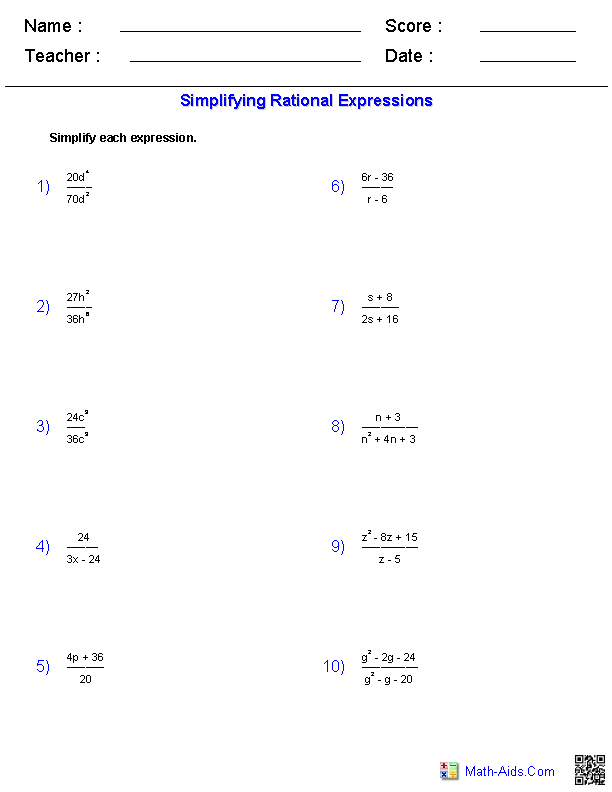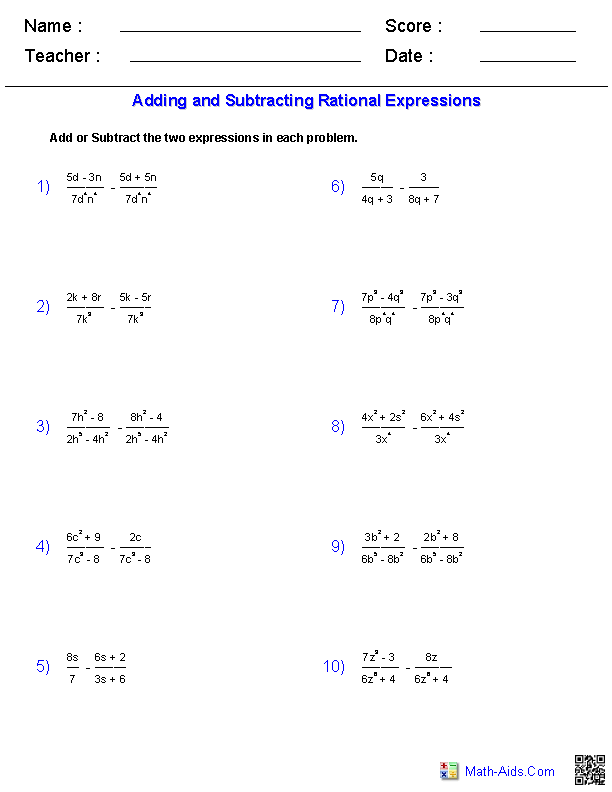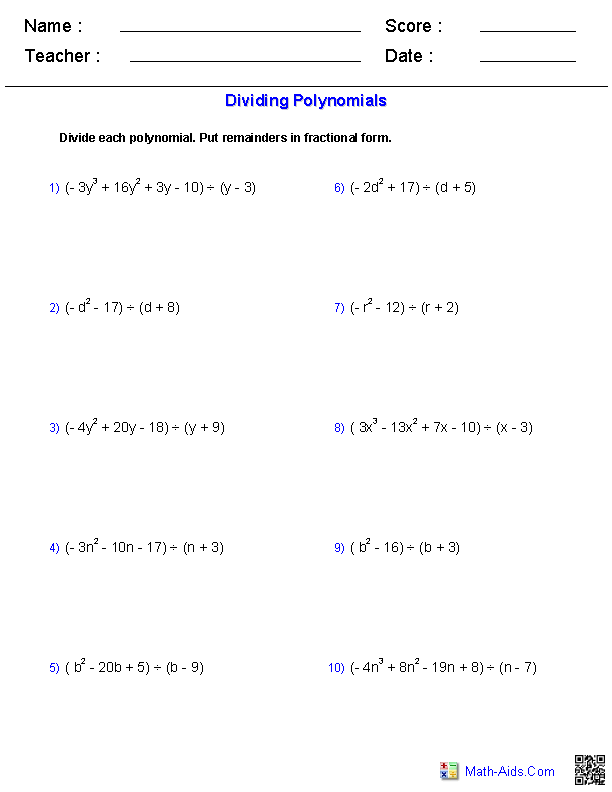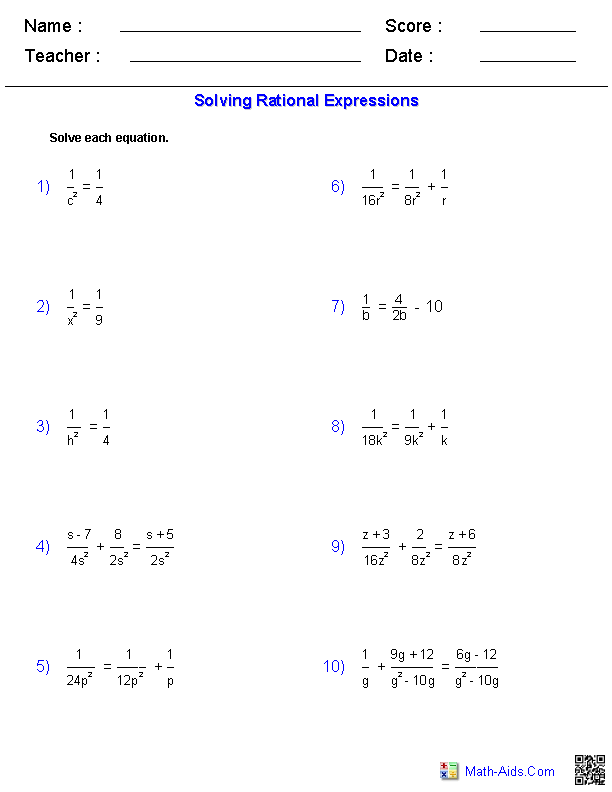#Algebra 1 Worksheets

## Rational Expressions Worksheets

Here is a graphic preview for all of the Rational Expressions Worksheets. You can select different variables to customize these Rational Expressions Worksheets for your needs. The Rational Expressions Worksheets are randomly created and will never repeat so you have an endless supply of quality Rational Expressions Worksheets to use in the classroom or at home. We have graphing quadratic functions, graphing quadratic inequalities, completing the square. We also have several solving quadratic equations by taking the square roots, factoring, with the quadratic formula, and by completing the square.

Our Rational Expressions Worksheets are free to download, easy to use, and very flexible.

These Rational Expressions Worksheets are a good resource for students in the 5th Grade through the 8th Grade.

Click here for a Detailed Description of all the Rational Expressions Worksheets.

## Quick Link for All Rational Expressions Worksheets

Click the image to be taken to that Rational Expressions Worksheets.

##### SimplifyingRational Expressions Worksheets##### Adding and SubtractingRational Expressions Worksheets##### MultiplyingRational Expressions Worksheets##### DividingRational Expressions Worksheets##### Dividing PolynomialsRational Expressions Worksheets##### Solving Rational EquationsRational Expressions WorksheetsRecommended Videos

## Detailed Description for All Rational Expressions Worksheets

Simplifying Radical Expressions Worksheets
These Rational Expressions Worksheets will produce problems for simplifying rational expressions. You may select what type of rational expression you want to use. These Rational Expressions Worksheets are a good resource for students in the 5th Grade through the 8th Grade.

Adding and Subtracting Rationals Expressions Worksheets
These Rational Expressions Worksheets will produce problems for adding and subtracting rational expressions. You may select what type of rational expression you want to use. These Rational Expressions Worksheets are a good resource for students in the 5th Grade through the 8th Grade.

Multiplying Rationals Expressions Worksheets
These Rational Expressions Worksheets will produce problems for multiplying rational expressions. You may select what type of rational expression you want to use. These Rational Expressions Worksheets are a good resource for students in the 5th Grade through the 8th Grade.

Dividing Rationals Expressions Worksheets
These Rational Expressions Worksheets will produce problems for dividing rational expressions. You may select what type of rational expression you want to use. These Rational Expressions Worksheets are a good resource for students in the 5th Grade through the 8th Grade.

Dividing Polynomials Worksheets
These Rational Expressions Worksheets will produce problems for dividing polynomials. You may select what type of polynomial you want to use. These Rational Expressions Worksheets are a good resource for students in the 5th Grade through the 8th Grade.

Solving Rational Expression Worksheets
These Rational Expressions Worksheets will produce problems for solving rational expressions. You may select what type of problem you want to use. These Rational Expressions Worksheets are a good resource for students in the 5th Grade through the 8th Grade.Math-Aids.Com.  All rights reserved. Algebra 1 - Rational Expressions Worksheets